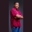Related Tags

swift
bitwise

# How to perform a bitwise AND operation in SwiftTheodore Kelechukwu Onyejiaku

## Overview

In Swift, we can perform a bitwise AND operation using the ampersand & operator. This operator requires two operands. It gets the binary representation of the operands, multiplies them, and returns the result as a decimal.

### Syntax

num1 & num2
Syntax for Bitwise AND(&) operation

### Parameters

• num1 and num2: These are the number values on which we want to perform a bitwise AND operation.

### Return value

This method returns the decimal equivalent of multiplying the bits of num1 and num2.

### Example

Let's look at the code below:

// create some values
var num1 = 3
var num2 = 2
var num3 = 100
var num4 = 20
var num5 = 1
var num6 = 0

// perform AND operation
print(num1 & num2) // 2
print(num3 & num4) // 4
print(num5 & num6) // 0
Performing AND operations in Swift

### Explanation

• Lines 2 to 7: We create some variables.
• Lines 10 to 12: We use the AND operator (&) and perform a bitwise AND operation on the values we created. We print the results to the console.

RELATED TAGS

swift
bitwise

CONTRIBUTORTheodore Kelechukwu Onyejiaku
RELATED COURSES

View all Courses

Keep Exploring

Learn in-demand tech skills in half the time